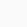# The Humble PEG Ratio: An Expert GuideAlex Frank

Updated on:

When it comes to evaluating the investment potential of a company, seasoned investors rely on a plethora of financial ratios and metrics. Among these, the PEG ratio stands out as a powerful tool for assessing a company’s growth prospects in relation to its current valuation. In this comprehensive guide, we will delve into the PEG ratio, exploring its definition, applications, formula, real-world examples, and comparing it with the Price-to-Earnings (P/E) ratio. We will also explore some variations and considerations to keep in mind while using this valuable metric.

## Understanding the PEG Ratio

The PEG ratio, which stands for Price/Earnings to Growth ratio, is a fundamental financial metric used by investors to assess a stock’s potential for growth relative to its current valuation. It combines two critical factors: the P/E ratio, which indicates the stock’s valuation, and the company’s expected earnings growth rate.

The PEG ratio provides a more comprehensive picture than the P/E ratio alone. It helps investors make more informed decisions by considering not only the price investors are willing to pay for a stock’s earnings (P/E) but also the expected growth of those earnings.

## Calculating the PEG Ratio

To calculate the PEG ratio, you need to follow these simple steps:

Step 1: Calculate the P/E Ratio

The P/E ratio is the price per share divided by the earnings per share (EPS). It is a widely-used valuation metric that represents how much investors are willing to pay for each dollar of a company’s earnings.

Step 2: Determine the Expected Earnings Growth Rate

You need to find the expected earnings growth rate of the company. This information can often be found in analysts’ reports, the company’s financial statements, or financial news sources.

Step 3: Calculate the PEG Ratio

Divide the P/E ratio (from Step 1) by the expected earnings growth rate (from Step 2). The formula for the PEG ratio is as follows:

\text{PEG Ratio} = \frac{\text{P/E Ratio}}{\text{Expected Earnings Growth Rate}}

## Interpreting the PEG Ratio

The PEG ratio provides a more nuanced perspective on a company’s valuation compared to the P/E ratio:

• A PEG ratio below 1 typically suggests that the stock may be undervalued, considering its growth prospects. Investors might view it as an attractive investment opportunity.
• A PEG ratio around 1 implies that the stock is reasonably valued based on its earnings growth expectations.
• A PEG ratio above 1 may indicate that the stock is overvalued relative to its growth potential. This could be a signal to exercise caution before investing.

## How PEG Ratio is Used

• Evaluating Growth Stocks: PEG ratio is particularly useful for assessing growth stocks. By incorporating the growth rate into the equation, investors can identify companies whose stock prices are not only justified by current earnings but also by their potential for future growth.
• Comparing Companies: Investors can use the PEG ratio to compare companies within the same industry. This comparison helps in determining which company offers better value concerning its growth prospects.
• Risk Assessment: PEG ratio aids in assessing the investment risk. A higher PEG ratio suggests that the market has high expectations for a company’s future earnings growth, which can be risky if the company fails to meet these expectations.

## A Comparative Analysis: PEG vs. P/E Ratio

Let’s compare the PEG ratio with the P/E ratio to understand their key differences and when to use each metric:

## Real-World Examples

Let’s look at a couple of real-world examples to illustrate how the PEG ratio can be applied in an investment context:

Example 1: Tech Company XYZ

Tech Company XYZ has a P/E ratio of 20 and is expected to have an earnings growth rate of 30% in the next year. Calculating the PEG ratio:

\text{PEG Ratio} = \frac{20}{30} = 0.67

In this case, the PEG ratio of 0.67 suggests that the stock may be undervalued relative to its growth potential, making it an attractive investment opportunity.

Example 2: Retailer ABC

Retailer ABC has a P/E ratio of 25 and is expected to have an earnings growth rate of 10% in the next year. Calculating the PEG ratio:

\text{PEG Ratio} = \frac{25}{10} = 2.5

Here, the PEG ratio of 2.5 indicates that the stock may be overvalued considering its growth prospects, which could lead to a more cautious investment approach.

## Variations on the PEG Ratio

Several variations and considerations exist when using the PEG ratio:

1. Forward PEG Ratio

The forward PEG ratio uses the estimated future earnings growth rate, providing a more up-to-date assessment of a company’s prospects. It’s essential to verify the accuracy of these estimates.

2. Trailing PEG Ratio

The trailing PEG ratio uses historical data for earnings and growth rates. While it provides a more concrete assessment, it may not reflect the company’s current situation accurately.

## Conclusion

The PEG ratio is a valuable tool for investors seeking to balance a stock’s valuation with its growth potential. By incorporating the P/E ratio and expected earnings growth rate, the PEG ratio offers a more comprehensive view of a company’s investment potential. However, it’s important to use this metric in conjunction with other financial analyses and consider variations, industry comparisons, and risk factors to make well-informed investment decisions.

Investing involves risks, and there is no one-size-fits-all formula for success. It’s crucial to conduct thorough research, diversify your portfolio, and seek professional advice when necessary. Understanding the PEG ratio is a significant step towards becoming a more informed and successful investor.